This is “Applications of Rational Equations”, section 7.6 from the book Beginning Algebra (v. 1.0). For details on it (including licensing), click here.

Has this book helped you? Consider passing it on:
Creative Commons supports free culture from music to education. Their licenses helped make this book available to you.
DonorsChoose.org helps people like you help teachers fund their classroom projects, from art supplies to books to calculators.

## 7.6 Applications of Rational Equations

### Learning Objectives

1. Solve applications involving relationships between real numbers.
2. Solve applications involving uniform motion (distance problems).
3. Solve work-rate applications.

## Number Problems

Recall that the reciprocalThe reciprocal of a nonzero number n is 1/n. of a nonzero number n is 1/n. For example, the reciprocal of 5 is 1/5 and 5 ⋅ 1/5 = 1. In this section, the applications will often involve the key word “reciprocal.” When this is the case, we will see that the algebraic setup results in a rational equation.

Example 1: A positive integer is 4 less than another. The sum of the reciprocals of the two positive integers is 10/21. Find the two integers.

Solution: Begin by assigning variables to the unknowns.Next, use the reciprocals $1n$ and $1n−4$ to translate the sentences into an algebraic equation.We can solve this rational expression by multiplying both sides of the equation by the least common denominator (LCD). In this case, the LCD is $21n(n−4)$.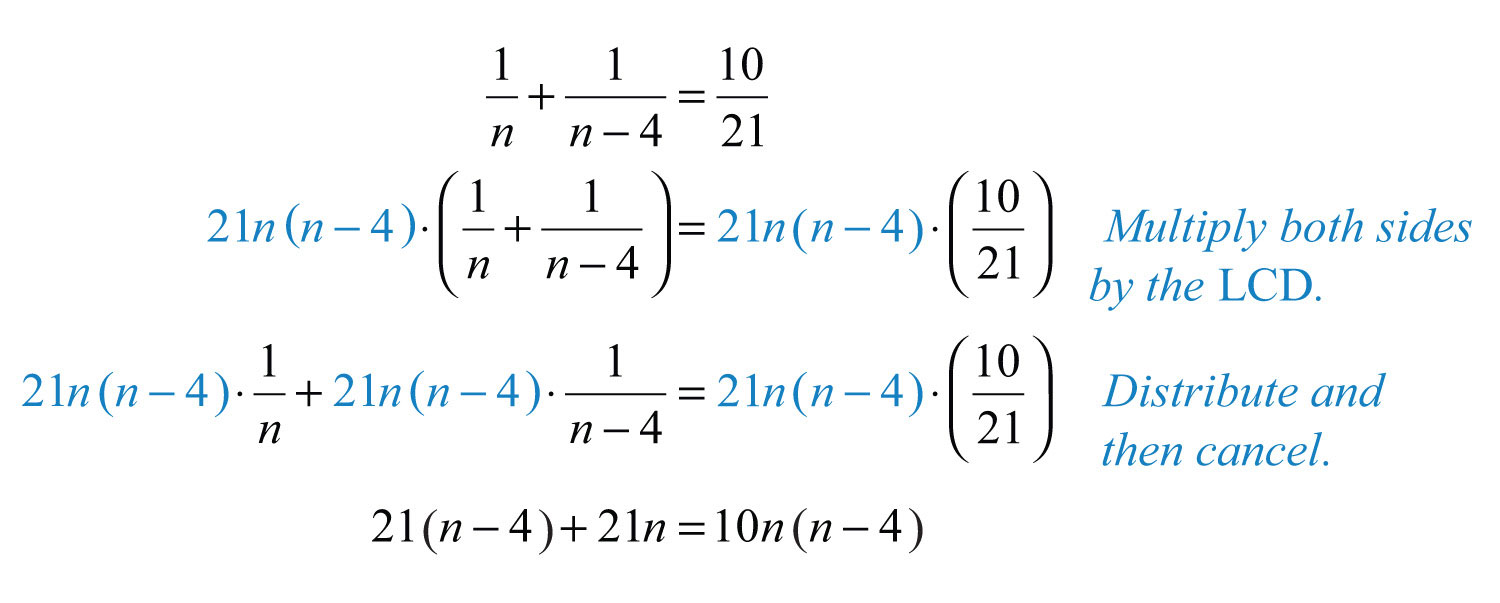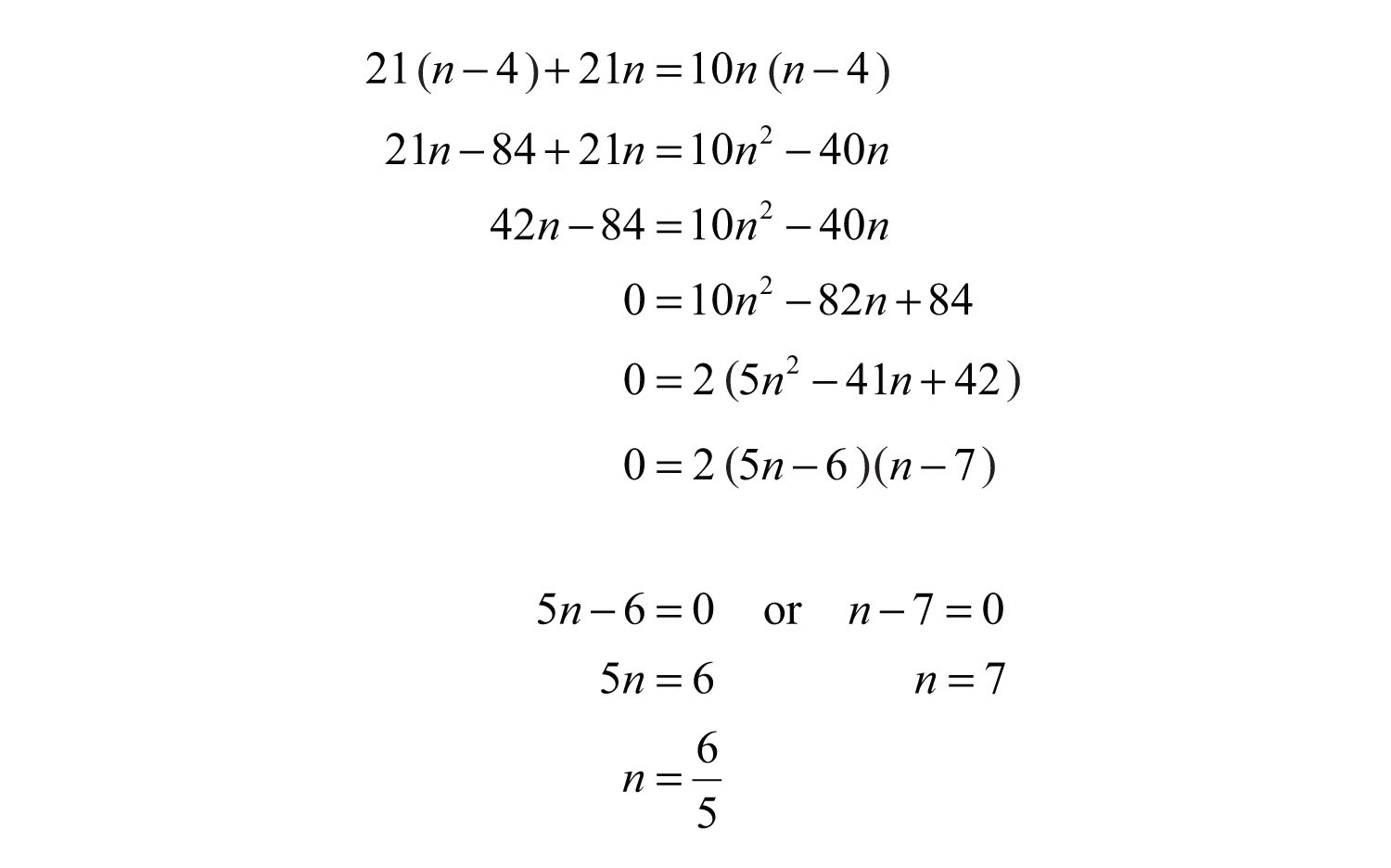The question calls for integers and the only integer solution is $n=7$. Hence disregard 6/5. Use the expression $n−4$ to find the smaller integer.Answer: The two positive integers are 3 and 7. The check is left to the reader.

Example 2: A positive integer is 4 less than another. If the reciprocal of the smaller integer is subtracted from twice the reciprocal of the larger, then the result is 1/30. Find the two integers.

Solution:Set up an algebraic equation.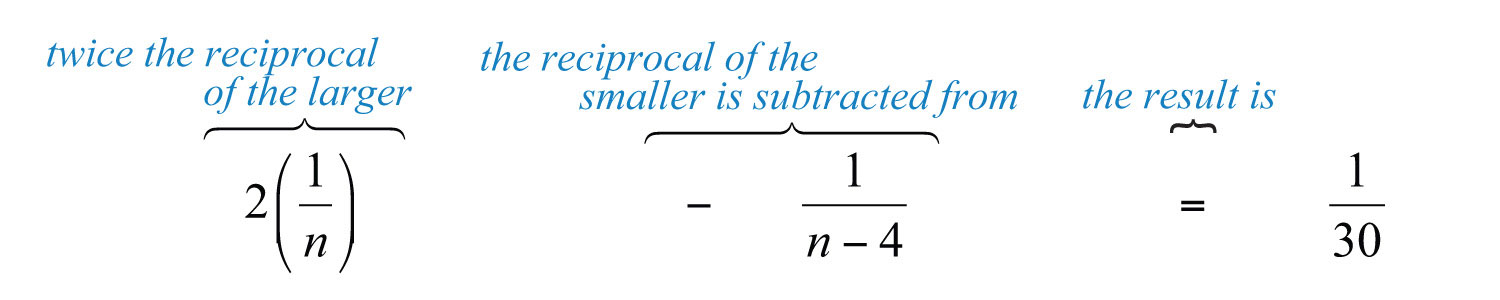Solve this rational expression by multiplying both sides by the LCD. The LCD is $30n(n−4)$.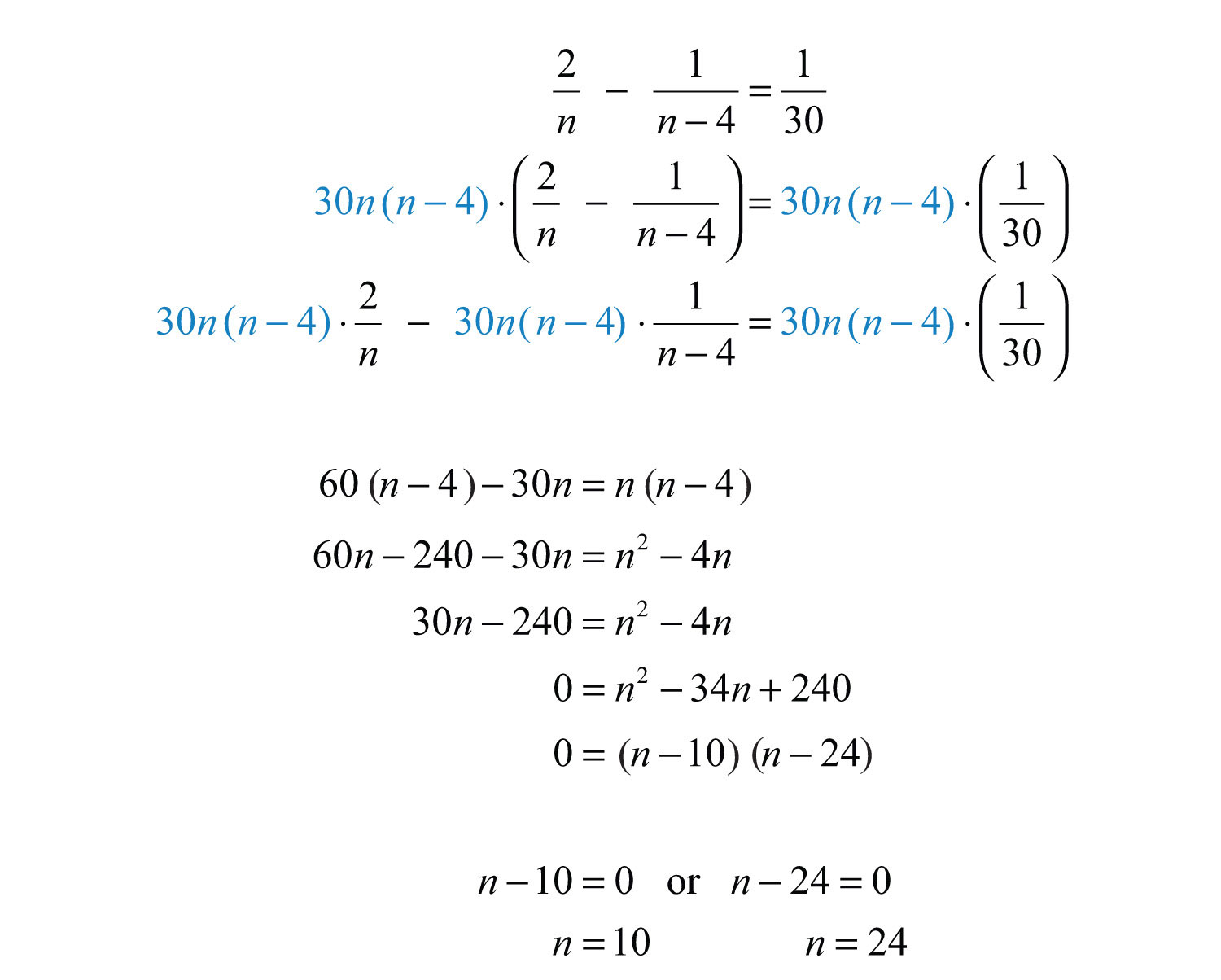Here we have two viable possibilities for the larger integer. For this reason, we will we have two solutions to this problem.As a check, perform the operations indicated in the problem.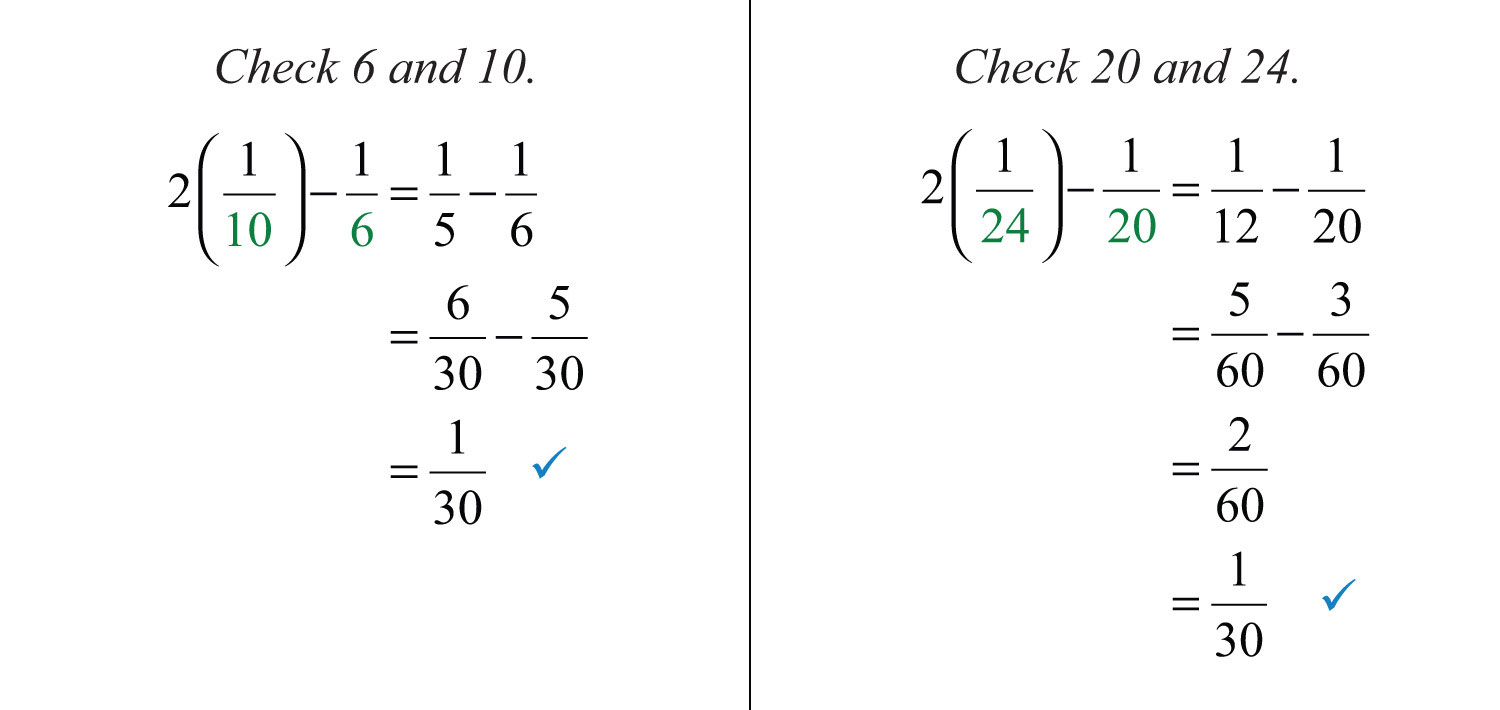Answer: Two sets of positive integers solve this problem: {6, 10} and {20, 24}.

Try this! The difference between the reciprocals of two consecutive positive odd integers is 2/15. Find the integers.

Answer: The integers are 3 and 5.

### Video Solution

(click to see video)

## Uniform Motion Problems

Uniform motionDescribed by the formula $D=rt$, where the distance, D, is given as the product of the average rate, r, and the time, t, traveled at that rate. problems, also referred to as distance problems, involve the formulawhere the distance, D, is given as the product of the average rate, r, and the time, t, traveled at that rate. If we divide both sides by the average rate, r, then we obtain the formulaFor this reason, when the unknown quantity is time, the algebraic setup for distance problems often results in a rational equation. Similarly, when the unknown quantity is the rate, the setup also may result in a rational equation.

We begin any uniform motion problem by first organizing our data with a chart. Use this information to set up an algebraic equation that models the application.

Example 5: Mary spent the first 120 miles of her road trip in traffic. When the traffic cleared, she was able to drive twice as fast for the remaining 300 miles. If the total trip took 9 hours, then how fast was she moving in traffic?

Solution: First, identify the unknown quantity and organize the data.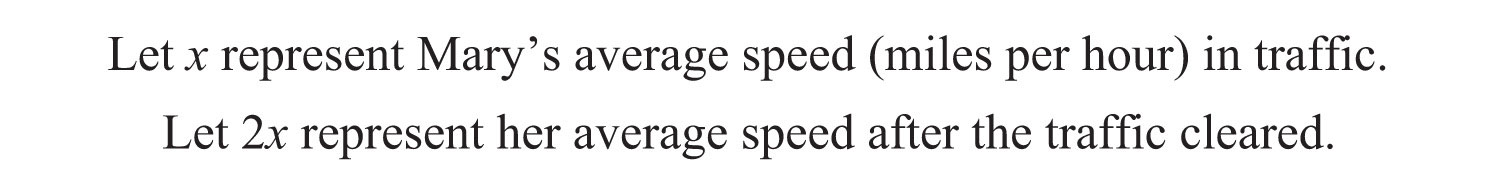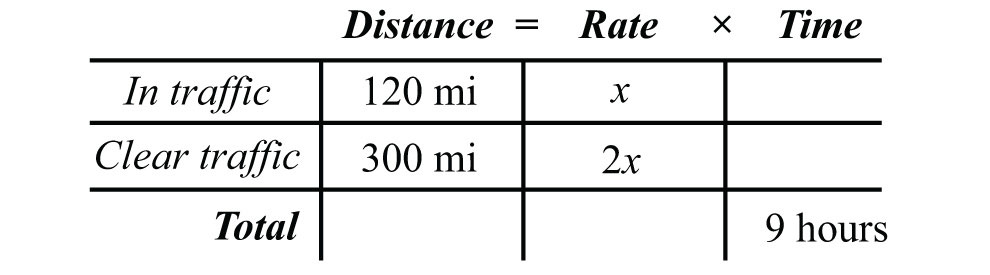To avoid introducing two more variables for the time column, use the formula $t=Dr$. Here the time for each leg of the trip is calculated as follows:Use these expressions to complete the chart.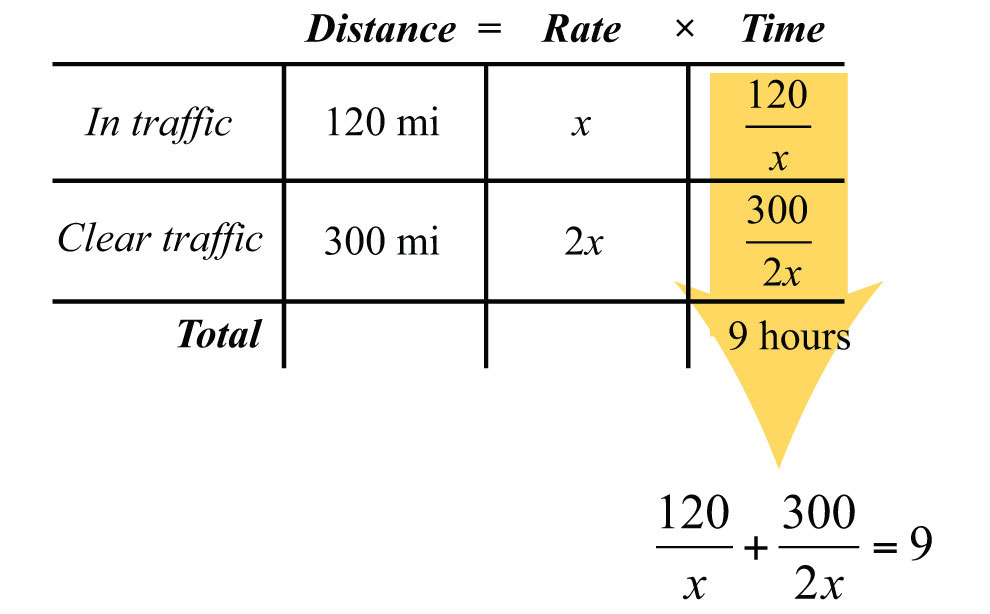The algebraic setup is defined by the time column. Add the times for each leg of the trip to obtain a total of 9 hours: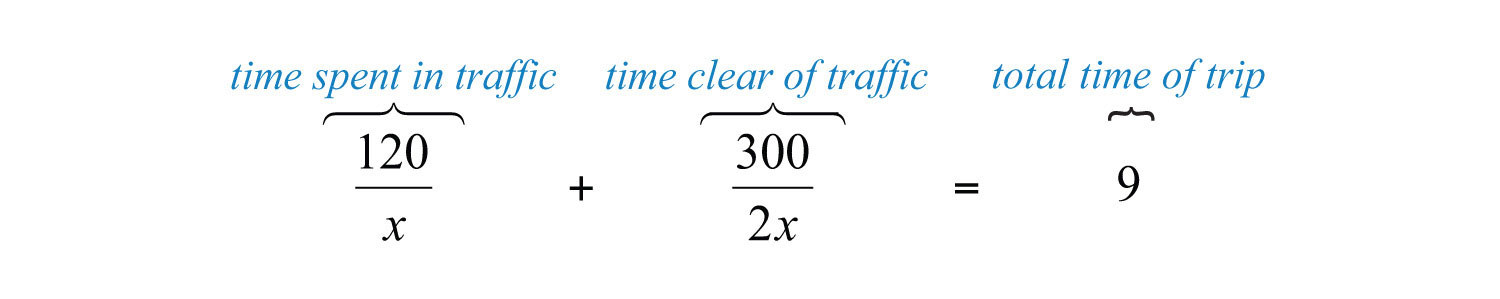We begin solving this equation by first multiplying both sides by the LCD, 2x.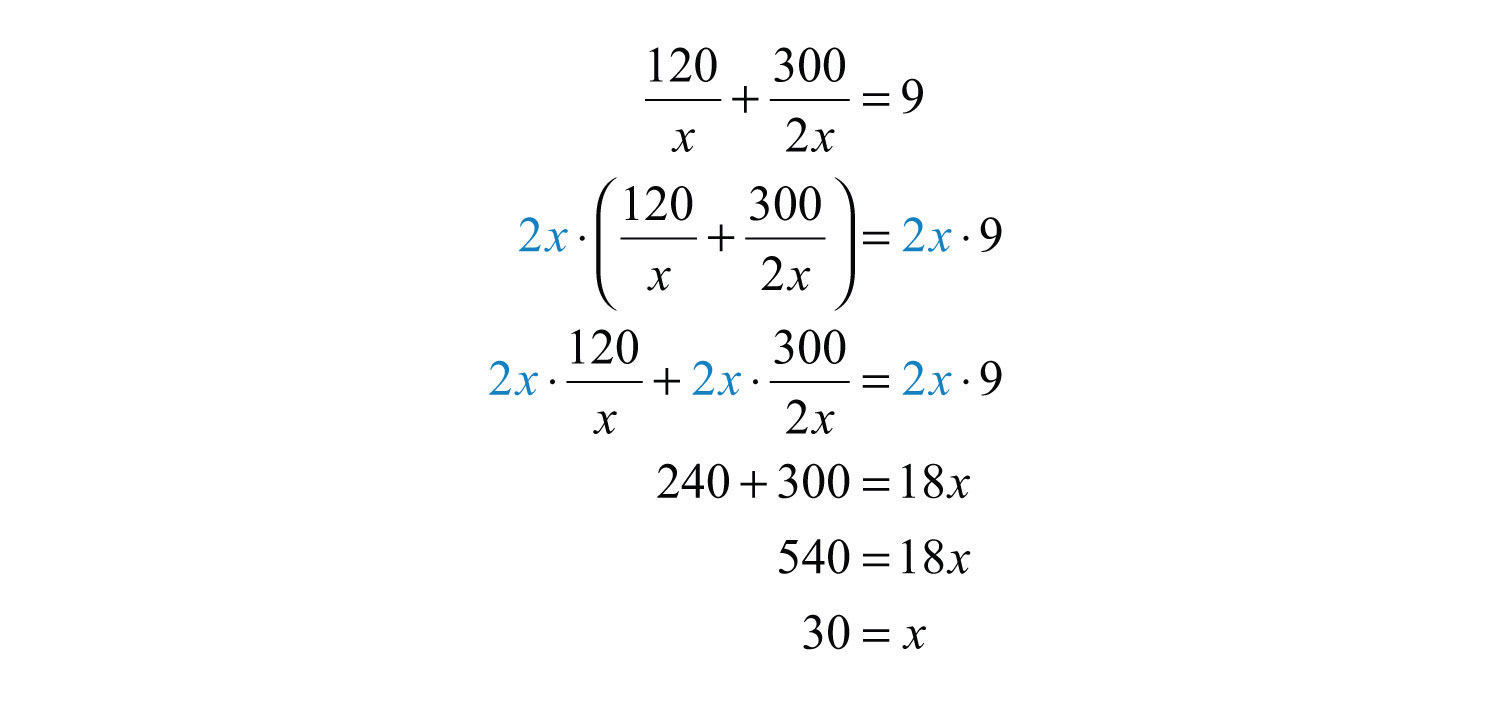Answer: Mary averaged 30 miles per hour in traffic.

Example 6: A passenger train can travel, on average, 20 miles per hour faster than a freight train. If the passenger train covers 390 miles in the same time it takes the freight train to cover 270 miles, then how fast is each train?

Solution: First, identify the unknown quantities and organize the data.Next, organize the given data in a chart.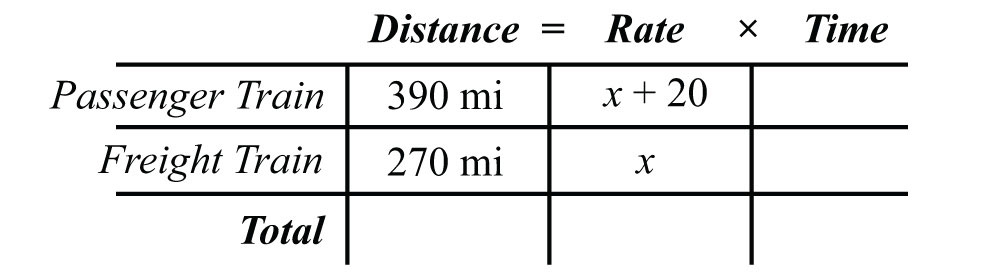Use the formula $t=Dr$ to fill in the time column for each train.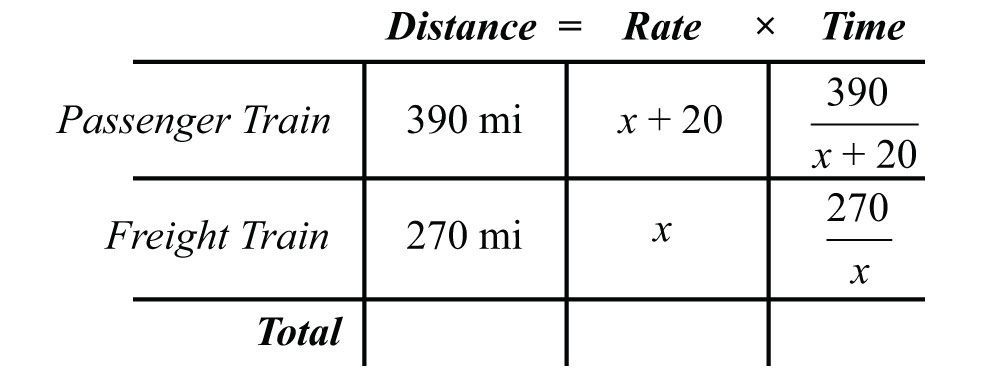Because the trains travel the same amount of time, finish the algebraic setup by equating the expressions that represent the times:Solve this equation by first multiplying both sides by the LCD, $x(x+20)$.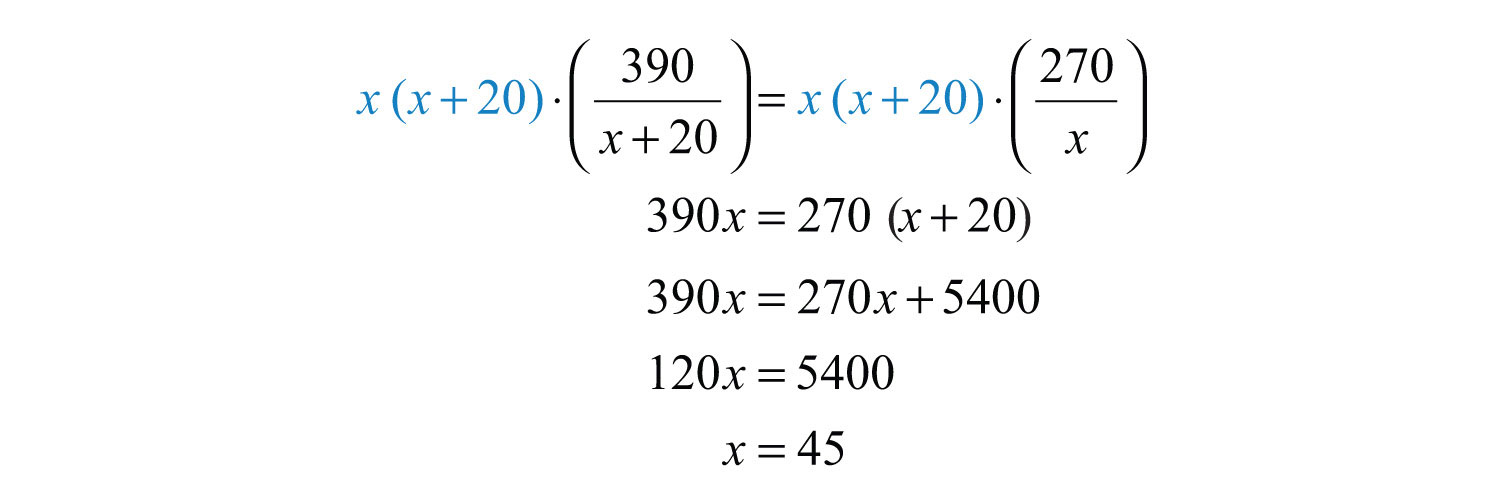Use x + 20 to find the speed of the passenger train.Answer: The speed of the passenger train is 65 miles per hour and the speed of the freight train is 45 miles per hour.

Example 7: Brett lives on the river 8 miles upstream from town. When the current is 2 miles per hour, he can row his boat downstream to town for supplies and back in 3 hours. What is his average rowing speed in still water?

Solution:Rowing downstream, the current increases his speed, and his rate is x + 2 miles per hour. Rowing upstream, the current decreases his speed, and his rate is x − 2 miles per hour. Begin by organizing the data in the following chart: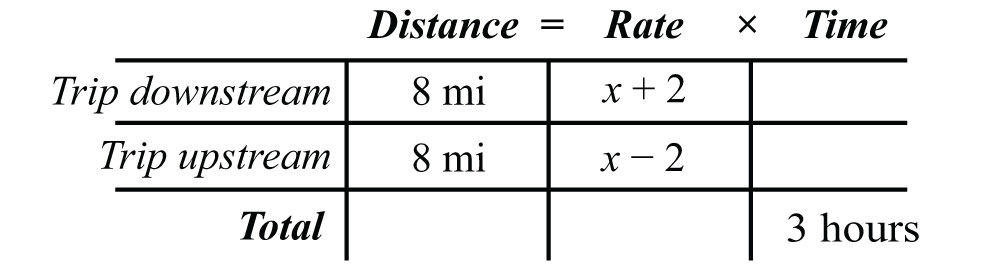Use the formula $t=Dr$ to fill in the time column for each leg of the trip.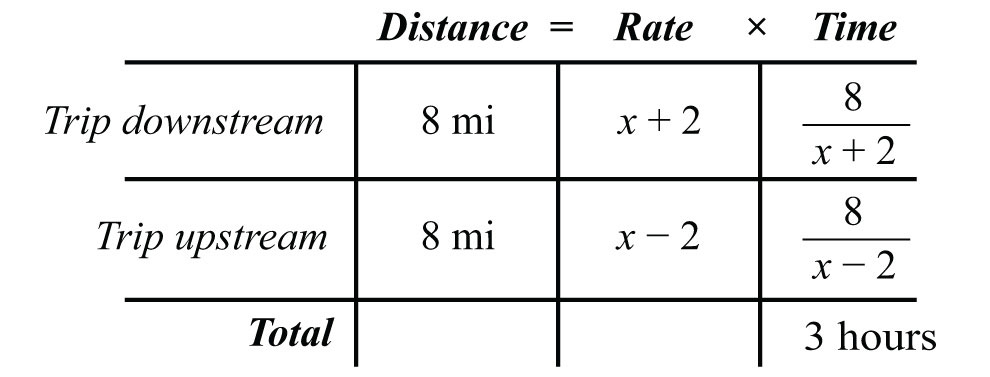The algebraic setup is defined by the time column. Add the times for each leg of the trip to obtain a total of 3 hours: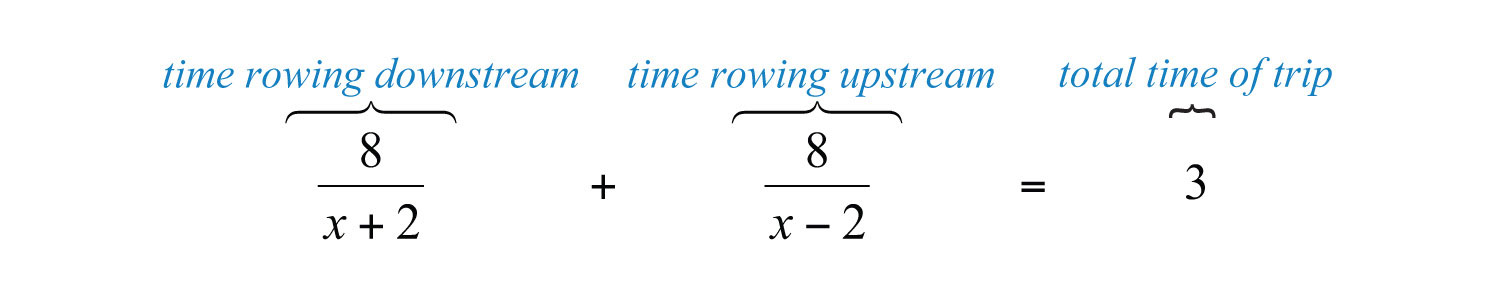Solve this equation by first multiplying both sides by the LCD, $(x+2)(x−2)$.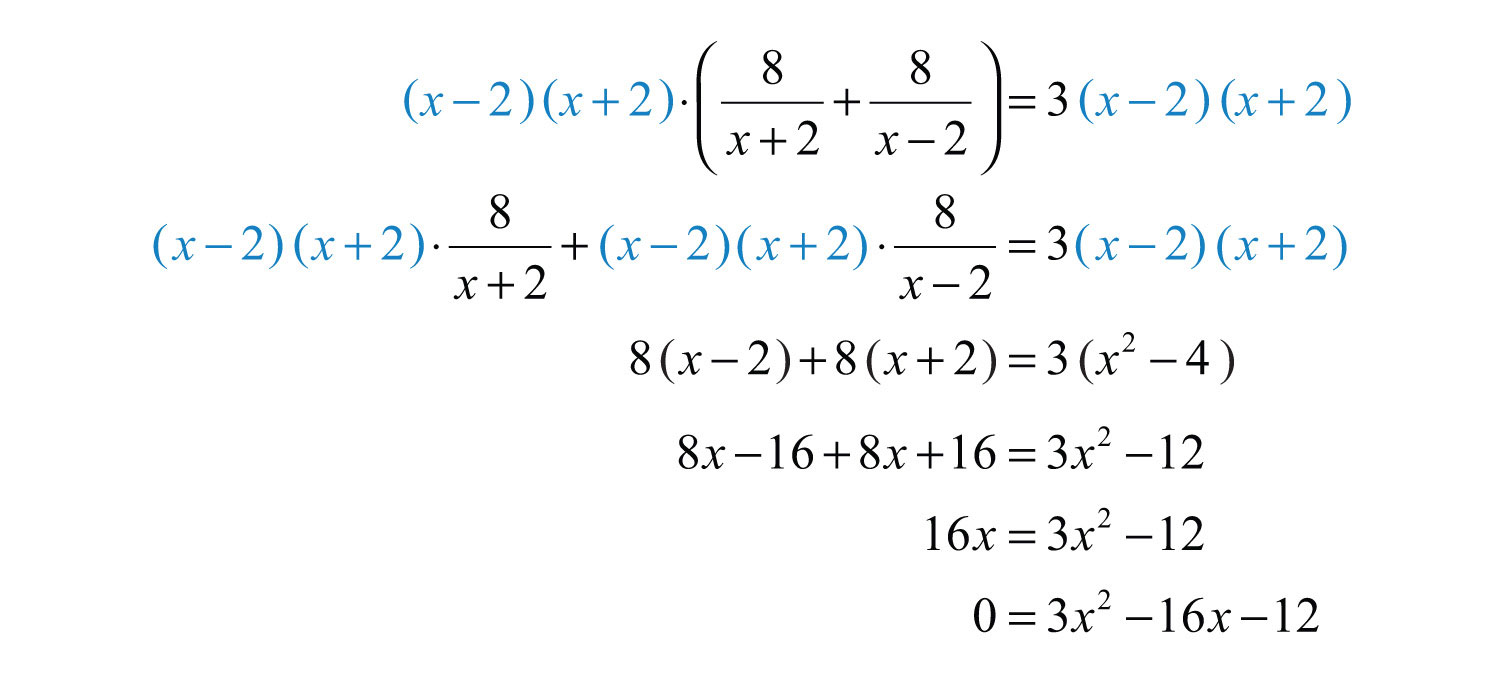Next, solve the resulting quadratic equation.Use only the positive solution, $x=6$ miles per hour.

Answer: His rowing speed is 6 miles per hour.

Try this! Dwayne drove 18 miles to the airport to pick up his father and then returned home. On the return trip he was able to drive an average of 15 miles per hour faster than he did on the trip there. If the total driving time was 1 hour, then what was his average speed driving to the airport?

Answer: His average speed driving to the airport was 30 miles per hour.

### Video Solution

(click to see video)

## Work-Rate Problems

The rate at which a task can be performed is called a work rateThe rate at which a task can be performed.. For example, if a painter can paint a room in 8 hours, then the task is to paint the room, and we can writeIn other words, the painter can complete $18$ of the task per hour. If he works for less than 8 hours, then he will perform a fraction of the task. For example,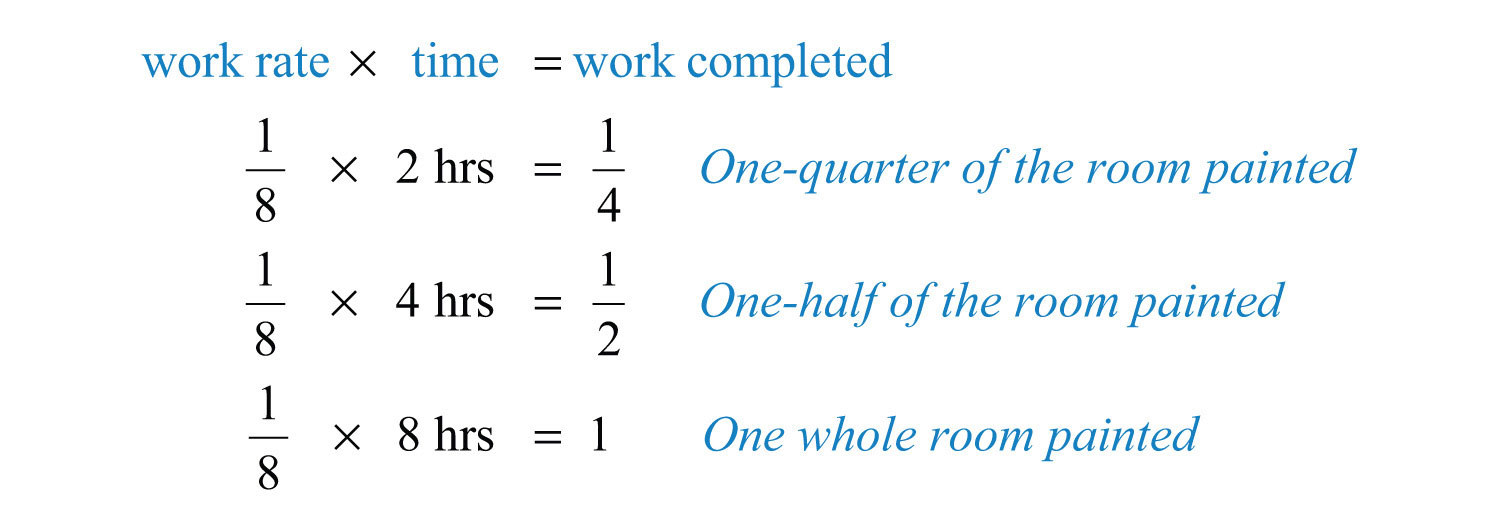Obtain the amount of the task completed by multiplying the work rate by the amount of time the painter works. Typically, work-rate problems involve people working together to complete tasks. When this is the case, we can organize the data in a chart, just as we have done with distance problems.

Suppose an apprentice painter can paint the same room by himself in 10 hours. Then we say that he can complete $110$ of the task per hour. Let t represent the time it takes both of the painters, working together, to paint the room.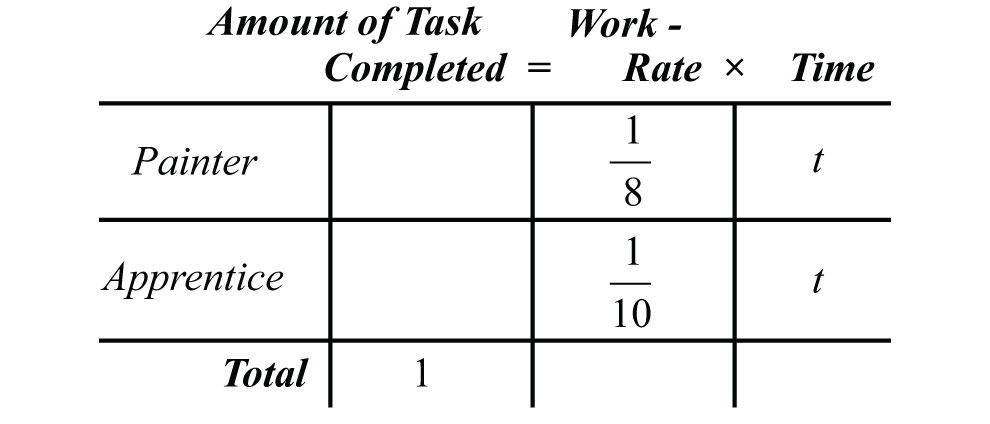To complete the chart, multiply the work rate by the time for each person. The portion of the room each can paint adds to a total of 1 task completed. This is represented by the equation obtained from the first column of the chart: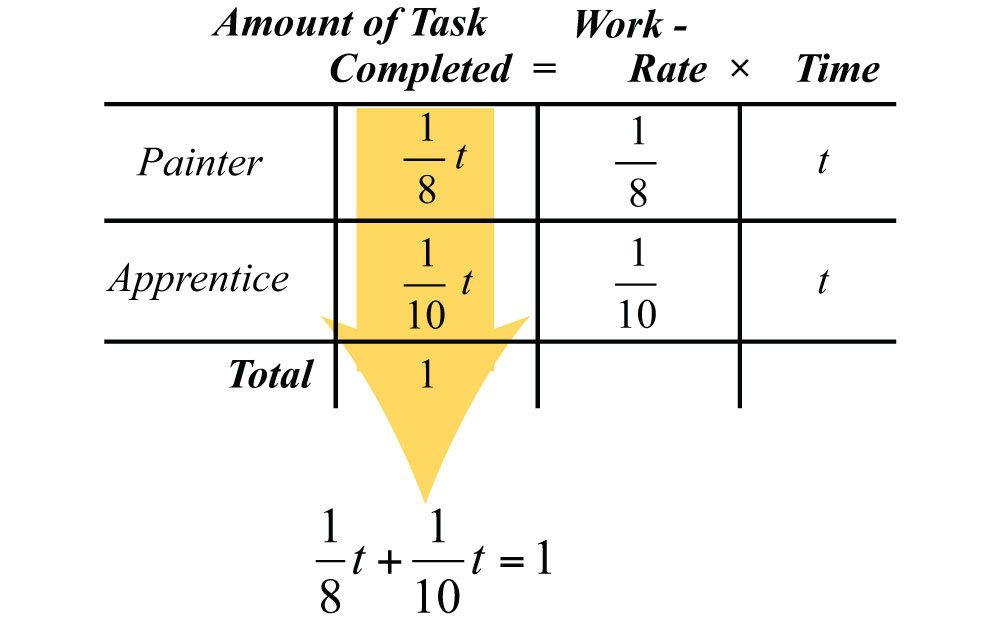This setup results in a rational equation that can be solved for t by multiplying both sides by the LCD, 40.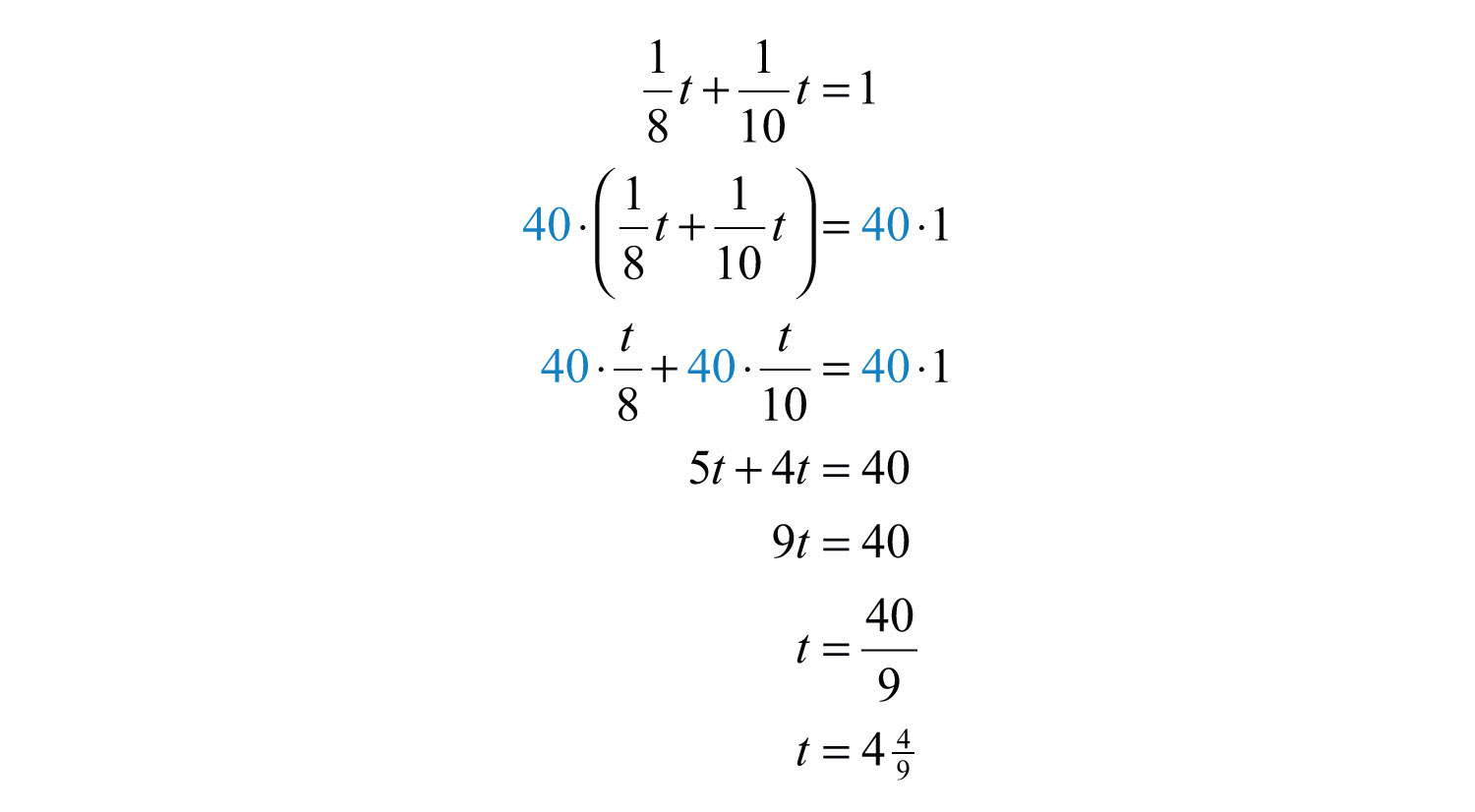Therefore, the two painters, working together, complete the task in $449$ hours.

In general, we have the following work-rate formula$1t1⋅t+1t2⋅t=1$, where $1t1$ and $1t2$ are the individual work rates and t is the time it takes to complete the task working together.:Here $1t1$ and $1t2$ are the individual work rates and t is the time it takes to complete one task working together. If we factor out the time, t, and then divide both sides by t, we obtain an equivalent work-rate formula:In summary, we have the following equivalent work-rate formulas: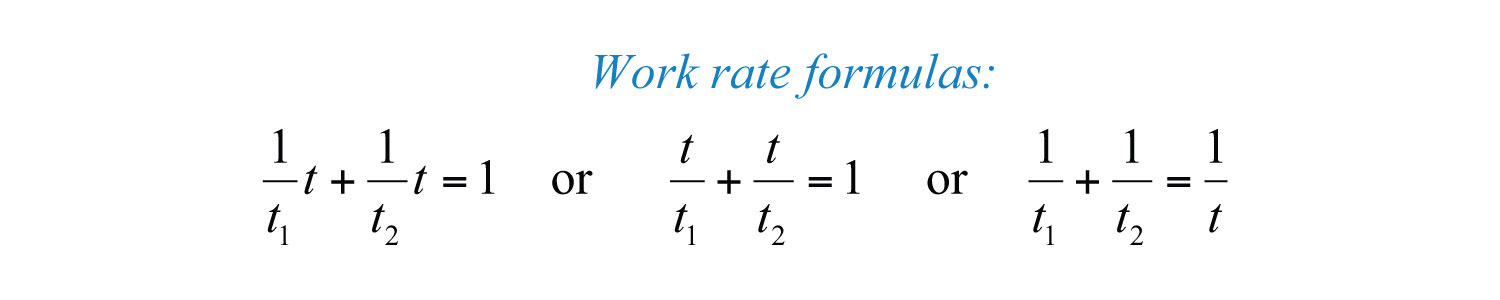Example 3: Working alone, Billy’s dad can complete the yard work in 3 hours. If Billy helps his dad, then the yard work takes 2 hours. How long would it take Billy working alone to complete the yard work?

Solution: The given information tells us that Billy’s dad has an individual work rate of $13$ task per hour. If we let x represent the time it takes Billy working alone to complete the yard work, then Billy’s individual work rate is $1x$, and we can write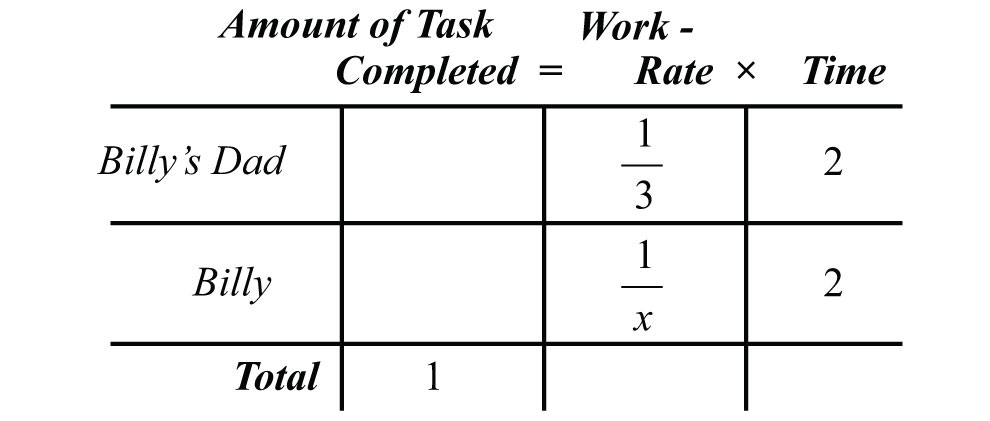Working together, they can complete the task in 2 hours. Multiply the individual work rates by 2 hours to fill in the chart.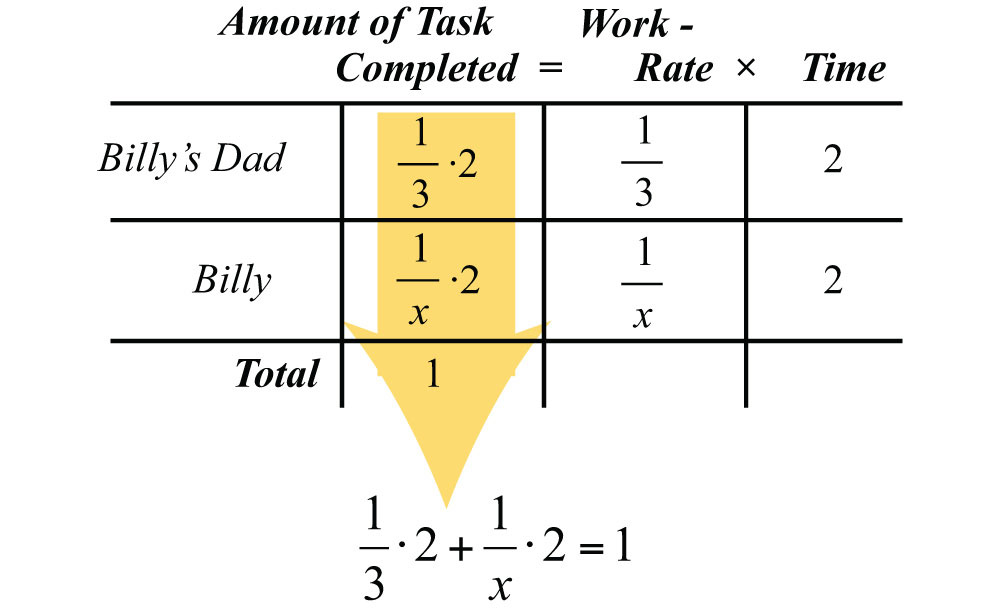The amount of the task each completes will total 1 completed task. To solve for x, we first multiply both sides by the LCD, 3x.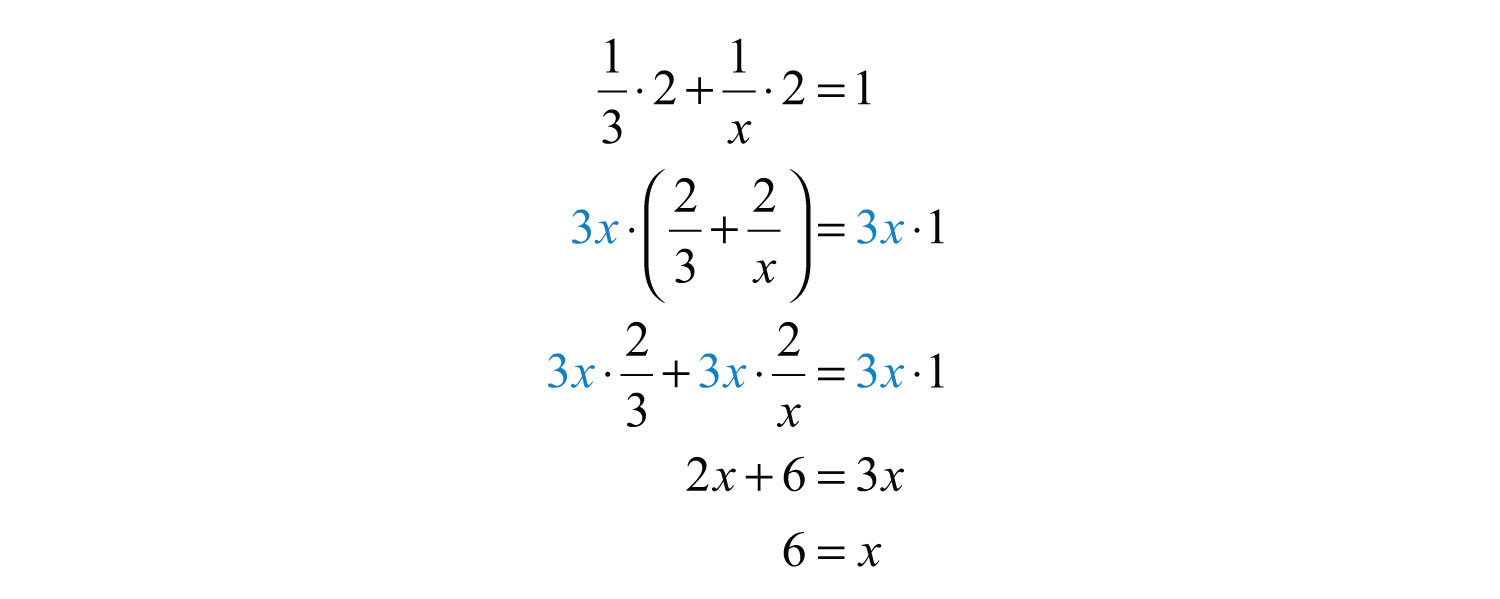Answer: It takes Billy 6 hours to complete the yard work alone.

Of course, the unit of time for the work rate need not always be in hours.

Example 4: Working together, two construction crews can build a shed in 5 days. Working separately, the less experienced crew takes twice as long to build a shed than the more experienced crew. Working separately, how long does it take each crew to build a shed?

Solution: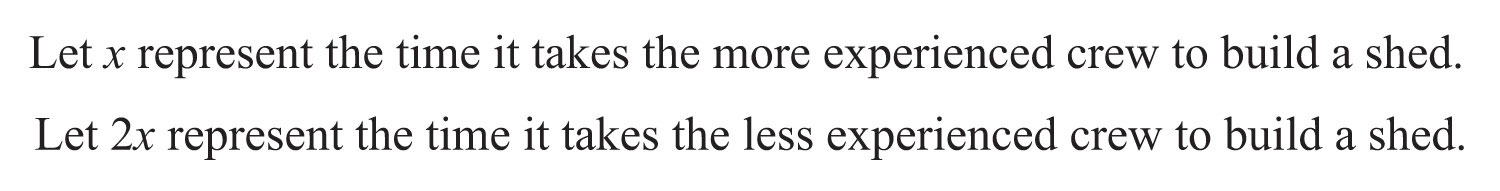Working together, the job is completed in 5 days. This gives the following setup: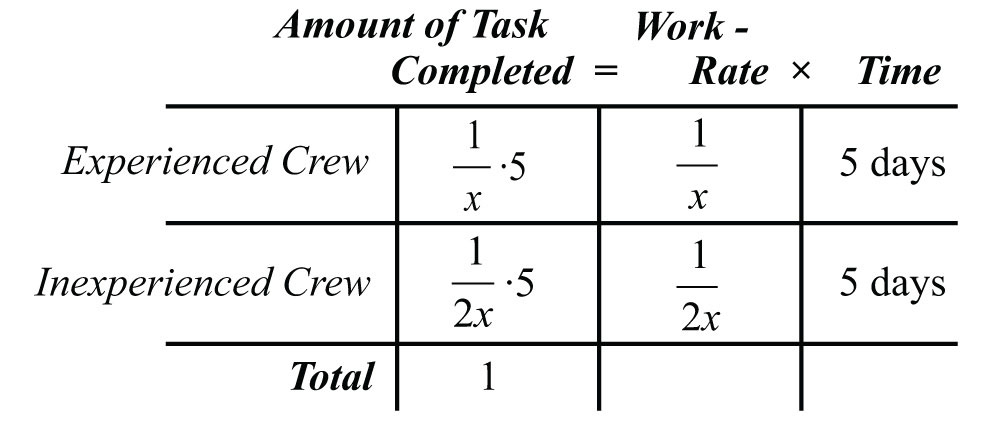The first column in the chart gives us an algebraic equation that models the problem:Solve the equation by multiplying both sides by 2x.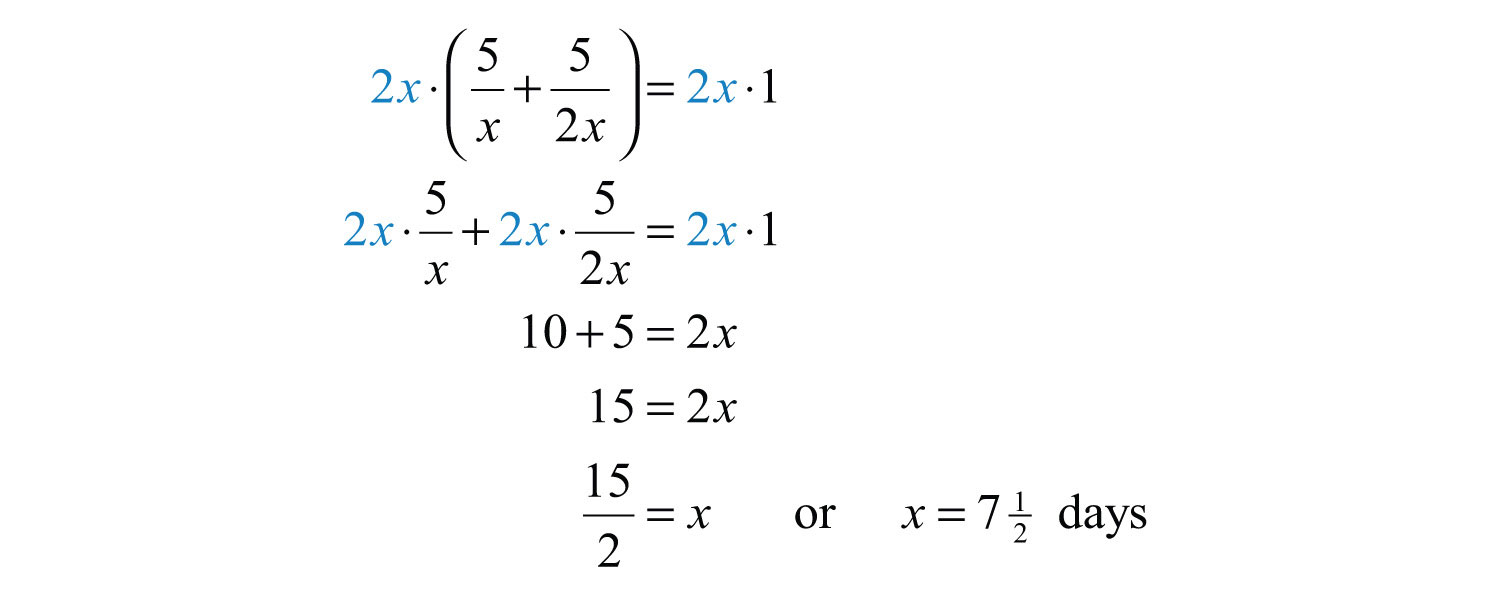To determine the time it takes the less experienced crew, we use 2x:Answer: Working separately, the experienced crew takes 7½ days to build a shed, and the less experienced crew takes 15 days to build a shed.

Try this! Joe’s garden hose fills the pool in 12 hours. His neighbor has a thinner hose that fills the pool in 15 hours. How long will it take to fill the pool using both hoses?

Answer: It will take both hoses $623$ hours to fill the pool.

### Video Solution

(click to see video)

### Key Takeaways

• In this section, all of the steps outlined for solving general word problems apply. Look for the new key word “reciprocal,” which indicates that you should write the quantity in the denominator of a fraction with numerator 1.
• When solving distance problems where the time element is unknown, use the equivalent form of the uniform motion formula, $t=Dr$, to avoid introducing more variables.
• When solving work-rate problems, multiply the individual work rate by the time to obtain the portion of the task completed. The sum of the portions of the task results in the total amount of work completed.

### Topic Exercises

Part A: Number Problems

Use algebra to solve the following applications.

1. A positive integer is twice another. The sum of the reciprocals of the two positive integers is 3/10. Find the two integers.

2. A positive integer is twice another. The sum of the reciprocals of the two positive integers is 3/12. Find the two integers.

3. A positive integer is twice another. The difference of the reciprocals of the two positive integers is 1/8. Find the two integers.

4. A positive integer is twice another. The difference of the reciprocals of the two positive integers is 1/18. Find the two integers.

5. A positive integer is 2 less than another. If the sum of the reciprocal of the smaller and twice the reciprocal of the larger is 5/12, then find the two integers.

6. A positive integer is 2 more than another. If the sum of the reciprocal of the smaller and twice the reciprocal of the larger is 17/35, then find the two integers.

7. The sum of the reciprocals of two consecutive positive even integers is 11/60. Find the two even integers.

8. The sum of the reciprocals of two consecutive positive odd integers is 16/63. Find the integers.

9. The difference of the reciprocals of two consecutive positive even integers is 1/24. Find the two even integers.

10. The difference of the reciprocals of two consecutive positive odd integers is 2/99. Find the integers.

11. If 3 times the reciprocal of the larger of two consecutive integers is subtracted from 2 times the reciprocal of the smaller, then the result is 1/2. Find the two integers.

12. If 3 times the reciprocal of the smaller of two consecutive integers is subtracted from 7 times the reciprocal of the larger, then the result is 1/2. Find the two integers.

13. A positive integer is 5 less than another. If the reciprocal of the smaller integer is subtracted from 3 times the reciprocal of the larger, then the result is 1/12. Find the two integers.

14. A positive integer is 6 less than another. If the reciprocal of the smaller integer is subtracted from 10 times the reciprocal of the larger, then the result is 3/7. Find the two integers.

Part B: Uniform Motion Problems

Use algebra to solve the following applications.

15. James can jog twice as fast as he can walk. He was able to jog the first 9 miles to his grandmother’s house, but then he tired and walked the remaining 1.5 miles. If the total trip took 2 hours, then what was his average jogging speed?

16. On a business trip, an executive traveled 720 miles by jet aircraft and then another 80 miles by helicopter. If the jet averaged 3 times the speed of the helicopter and the total trip took 4 hours, then what was the average speed of the jet?

17. Sally was able to drive an average of 20 miles per hour faster in her car after the traffic cleared. She drove 23 miles in traffic before it cleared and then drove another 99 miles. If the total trip took 2 hours, then what was her average speed in traffic?

18. Harry traveled 15 miles on the bus and then another 72 miles on a train. If the train was 18 miles per hour faster than the bus and the total trip took 2 hours, then what was the average speed of the train?

19. A bus averages 6 miles per hour faster than a trolley. If the bus travels 90 miles in the same time it takes the trolley to travel 75 miles, then what is the speed of each?

20. A passenger car averages 16 miles per hour faster than the bus. If the bus travels 56 miles in the same time it takes the passenger car to travel 84 miles, then what is the speed of each?

21. A light aircraft travels 2 miles per hour less than twice as fast as a passenger car. If the passenger car can travel 231 miles in the same time it takes the aircraft to travel 455 miles, then what is the average speed of each?

22. Mary can run 1 mile per hour more than twice as fast as Bill can walk. If Bill can walk 3 miles in the same time it takes Mary to run 7.2 miles, then what is Bill’s average walking speed?

23. An airplane traveling with a 20-mile-per-hour tailwind covers 270 miles. On the return trip against the wind, it covers 190 miles in the same amount of time. What is the speed of the airplane in still air?

24. A jet airliner traveling with a 30-mile-per-hour tailwind covers 525 miles in the same amount of time it is able to travel 495 miles after the tailwind eases to 10 miles per hour. What is the speed of the airliner in still air?

25. A boat averages 16 miles per hour in still water. With the current, the boat can travel 95 miles in the same time it travels 65 miles against it. What is the speed of the current?

26. A river tour boat averages 7 miles per hour in still water. If the total 24-mile tour downriver and 24 miles back takes 7 hours, then how fast is the river current?

27. If the river current flows at an average 3 miles per hour, then a tour boat makes the 9-mile tour downstream with the current and back the 9 miles against the current in 4 hours. What is the average speed of the boat in still water?

28. Jane rowed her canoe against a 1-mile-per-hour current upstream 12 miles and then returned the 12 miles back downstream. If the total trip took 5 hours, then at what speed can Jane row in still water?

29. Jose drove 15 miles to pick up his sister and then returned home. On the return trip, he was able to average 15 miles per hour faster than he did on the trip to pick her up. If the total trip took 1 hour, then what was Jose’s average speed on the return trip?

30. Barry drove the 24 miles to town and then back in 1 hour. On the return trip, he was able to average 14 miles per hour faster than he averaged on the trip to town. What was his average speed on the trip to town?

31. Jerry paddled his kayak upstream against a 1-mile-per-hour current for 12 miles. The return trip downstream with the 1-mile-per-hour current took 1 hour less time. How fast can Jerry paddle the kayak in still water?

32. It takes a light aircraft 1 hour more time to fly 360 miles against a 30-mile-per-hour headwind than it does to fly the same distance with it. What is the speed of the aircraft in calm air?

Part C: Work-Rate Problems

Use algebra to solve the following applications.

33. James can paint the office by himself in 7 hours. Manny paints the office in 10 hours. How long will it take them to paint the office working together?

34. Barry can lay a brick driveway by himself in 12 hours. Robert does the same job in 10 hours. How long will it take them to lay the brick driveway working together?

35. Jerry can detail a car by himself in 50 minutes. Sally does the same job in 1 hour. How long will it take them to detail a car working together?

36. Jose can build a small shed by himself in 26 hours. Alex builds the same small shed in 2 days. How long would it take them to build the shed working together?

37. Allison can complete a sales route by herself in 6 hours. Working with an associate, she completes the route in 4 hours. How long would it take her associate to complete the route by herself?

38. James can prepare and paint a house by himself in 5 days. Working with his brother, Bryan, they can do it in 3 days. How long would it take Bryan to prepare and paint the house by himself?

39. Joe can assemble a computer by himself in 1 hour. Working with an assistant, he can assemble a computer in 40 minutes. How long would it take his assistant to assemble a computer working alone?

40. The teacher’s assistant can grade class homework assignments by herself in 1 hour. If the teacher helps, then the grading can be completed in 20 minutes. How long would it take the teacher to grade the papers working alone?

41. A larger pipe fills a water tank twice as fast as a smaller pipe. When both pipes are used, they fill the tank in 5 hours. If the larger pipe is left off, then how long would it take the smaller pipe to fill the tank?

42. A newer printer can print twice as fast as an older printer. If both printers working together can print a batch of flyers in 45 minutes, then how long would it take the newer printer to print the batch working alone?

43. Working alone, Henry takes 9 hours longer than Mary to clean the carpets in the entire office. Working together, they clean the carpets in 6 hours. How long would it take Mary to clean the office carpets if Henry were not there to help?

44. Working alone, Monique takes 4 hours longer than Audrey to record the inventory of the entire shop. Working together, they take inventory in 1.5 hours. How long would it take Audrey to record the inventory working alone?

45. Jerry can lay a tile floor in 3 hours less time than Jake. If they work together, the floor takes 2 hours. How long would it take Jerry to lay the floor by himself?

46. Jeremy can build a model airplane in 5 hours less time than his brother. Working together, they need 6 hours to build the plane. How long would it take Jeremy to build the model airplane working alone?

47. Harry can paint a shed by himself in 6 hours. Jeremy can paint the same shed by himself in 8 hours. How long will it take them to paint two sheds working together?

48. Joe assembles a computer by himself in 1 hour. Working with an assistant, he can assemble 10 computers in 6 hours. How long would it take his assistant to assemble 1 computer working alone?

49. Jerry can lay a tile floor in 3 hours, and his assistant can do the same job in 4 hours. If Jerry starts the job and his assistant joins him 1 hour later, then how long will it take to lay the floor?

50. Working alone, Monique takes 6 hours to record the inventory of the entire shop, while it takes Audrey only 4 hours to do the same job. How long will it take them working together if Monique leaves 2 hours early?

1: {5, 10}

3: {4, 8}

5: {6, 8}

7: {10, 12}

9: {6, 8}

11: {1, 2} or {−4, −3}

13: {4, 9} or {15, 20}

15: 6 miles per hour

17: 46 miles per hour

19: Trolley: 30 miles per hour; bus: 36 miles per hour

21: Passenger car: 66 miles per hour; aircraft: 130 miles per hour

23: 115 miles per hour

25: 3 miles per hour

27: 6 miles per hour

29: 40 miles per hour

31: 5 miles per hour

33: $4217$ hours

35: $27311$ minutes

37: 12 hours

39: 2 hours

41: 15 hours

43: 9 hours

45: 3 hours

47: $667$ hours

49: $217$ hours